# Discount Printer Template Syntax

So I am getting into templates and need help understanding some of the syntax. Firstly I will post two sections as currently used:

``````{DISCOUNTS}
<J00>Discounts:|[=F(TN('{ORDER TAG TOTAL:Discount}')+TN('{ORDER TAG TOTAL:VIP Discount}')+TN('{ORDER TAG TOTAL:HH Discount}'))]
<J00>TOTAL SAVINGS:|[=F(-1*(TN('{ORDER TAG TOTAL:Discount}')+TN('{ORDER TAG TOTAL:VIP Discount}')+TN('{ORDER TAG TOTAL:HH Discount}'))+TN('{ORDER STATE TOTAL:Gift}'))]
``````

AND (stock standard)

``````[DISCOUNTS]
<J00>{CALCULATION NAME} %{CALCULATION AMOUNT}|{CALCULATION TOTAL}
``````

My results were: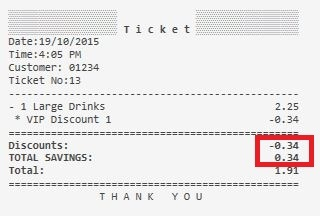If zero obviously without the square brackets “[ ]” as shown in QMcKay tutorial I will get: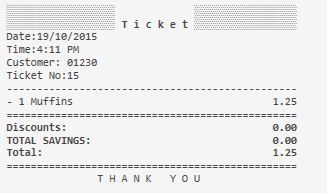Questions:
If I use square brackets I get sample ticket below even if there are amounts in VIP discount?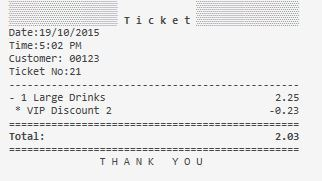``````[<J10>Total Gift:|{ORDER STATE TOTAL:Gift}]
[<J00>Discounts:|[=F(TN('{ORDER TAG TOTAL:Discount}')+TN('{ORDER TAG TOTAL:VIP Discount}')+TN('{ORDER TAG TOTAL:HH Discount}'))]]
[<J00>TOTAL SAVINGS:|[=F(-1*(TN('{ORDER TAG TOTAL:Discount}')+TN('{ORDER TAG TOTAL:VIP Discount}')+TN('{ORDER TAG TOTAL:HH Discount}'))+TN('{ORDER STATE TOTAL:Gift}'))]]
``````

In my preview - the last bracket on the bottom 2 lines is in a different font size??

Another Question:
How does the [DISCOUNT] section relate to the {DISCOUNTS} Tag?
I can see how it formats the line if I use the standard settlement discount button like:
DIscount %10 … -0.84
But I see no relevance to the VIP & HH sections in my template.

Also @emre I seem to manage to get no Help or Preview as shown below, Is this correct, as I need to log out and back in to get the preview back?

[DISCOUNTS] is the template for {DISCOUNTS} its really that simple. Anything under [DISCOUNTS] will be displayed wherever you put {DISCOUNTS} in your printer template. There are tags specific for Discounts just like there are tags specific for Services and Taxes. But you can also display other things if used inside the bracketed Template.

So is this by design or my screw up. If I add another line in the {DISCOUNTS} tag like below as SpecialV: I get a result whe the lines are enclosed with “[ ] if I only use VIP Discount Tag which had the value?:”

``````{DISCOUNTS}
[<J00>SpecialV:|[=F(TN('{ORDER TAG TOTAL:VIP Discount}'),'0.00')]]
[<J00>Specials:|[=F(TN('{ORDER TAG TOTAL:Discount}')+TN('{ORDER TAG TOTAL:VIP Discount}')+TN('{ORDER TAG TOTAL:HH Discount}'),'0.00')]]
[<J00>TOTAL SAVINGS:|[=F(-1*(TN('{ORDER TAG TOTAL:Discount}')+TN('{ORDER TAG TOTAL:VIP Discount}')+TN('{ORDER TAG TOTAL:HH Discount}'))+TN('{ORDER STATE TOTAL:Gift}'))]]
``````

Result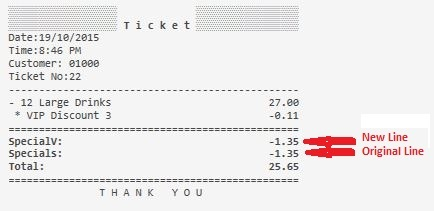Note: I only added the formatting of “0.00” as I thought maybe because the result was less than 1 (<1) so also built up the value as well.

I dont understand the problem? Whats wrong about it?

I wish to keep the line “Specials:” which has the sum of Discount (standard) + VIP Discount + HH Discount but if I use the “[ ]” brackets it prints nothing? If I remove the brackets it will print “0” values…

Its only printing now because I have added the line “SpecialV:” which places the VIP discount on a separate line? SO not sure if its my ignorance. Yes see your reply below but if I remove the SpecialV line it seems not to calculate.

I am confused you showed it with brackets and then a printout of it working? EDIT: I see what your saying.

Why not just put it under [DISCOUNTS] and simply use {DISCOUNTS} in template?

Ok that’s I think may be the issue as from the V5 Tutorial Q did not say where to place the code… So I think it has to be calculated first in the [DISCOUNT] layout section before using the {DISCOUNT} Tag. That’s why I asked before I do not see the relationship.

Like why does the {DISCOUNT} Tag have a line:

``````[<J10>Total Gift:|{ORDER STATE TOTAL:Gift}]
``````

Why is that not in the [DISCOUNT] section? I will get this as it my first attempt…

Ok moving it to [DISCOUNTS] did not work.

It doesnt have to be, but [DISCOUNT] is a layout template. It makes your Printer Template easier to read and modify later and easier to spot problems if you use appropriate Layout Template sections.

For example if something messes up with Discounts you know that all Discount Relate Tags are under [DISCOUNTS] layout so you can go straight to it vs having to look through your template to find all related tags.

You could get rid of layout sections completely and template will work fine but it will make it VERY difficult to decipher various sections. Some Layout sections contain specific tags for those sections… Example if its Order Related tags then it will only work under [ORDERS] section.

Thanks kendah, this is the simple thing I was trying to implement into the standard ticket template:

Just not sure where to place it OR get it to work…

Does it explains how Section Tags (`[xxx]`) works?

1 Like

Yes that is now becoming clear. The confusion was 2 things:

1. Using a custom format other than the [template] such as `[<J10>Total Gift:|{ORDER STATE TOTAL:Gift}]` used in the {DISCOUNT} section in the sample Ticket Template.

2. The other unsolved is why if I am using a custom format in the {DISCOUNT} section I need to list the different {ORDER TAG TOTAL:x} on a separate line before it will print on a line with all {ORDER TAG TOTAL:x’s} added together such as Q’s “Discounts” line?

3. The “TOTAL SAVINGS” line just has not appeared as yet…

Item (2) & (3) is the things I do not get.

{DISCOUNT} section used to list calculation templates used as discounts (configured to decrease ticket amount). The term confuses you but even it named as Discount what you’re listing there is Order Tags. {ORDER TAG TOTAL:X} is a base template (ticket) tag that returns total price of order tags named as x. As you use these order tags as discounts you’re referring them with discount name. That has no relationship with {DISCOUNT} or [DISCOUNT] sections.

2 Likes

This is not used in [DISCOUNT] section. It appears just after what {DISCOUNT} tag prints.

How am I doing that, in my syntax or just what I am typing here? Ok I see you have replied…
Yes I was slowing getting that by sheer volume of reading threads!

So the reason why Order Tags used for VIP discount needed to be listed separately? OR without the “[ ]” at each end (omit blank lines).

Sorry pauln I may have confused you I did poor job explaining it.

No kendash any help is always appreciated! I just needing to “brake the back of this” so to speak and I am getting my head around the concepts. Just difficult starting as you do not know if you (me) have just stuffed something up or the syntax is wrong.

Lol sorry @pauln my English really suckz. Let me explain once more.

{DISCOUNT} is a place holder for Calculation Templates that decreases ticket amount. What appears after this tag will print after discount list.
[DISCOUNT] and what appears under that until next [X] section is… the template we’ll use to render calculation templates.

What you’re doing does have 0 relationship with Discounts (as a ticket template feature). You’re using (what I mean with you is your implementation) Order Tags as Discounts. So you’re calling these order tags as `Discount`. I think that confuses you. That was my point. Naming something as `Discount` does not mean it relates with Calculation Templates. Think it like I can create a ticket tag and name it as discount to store some amount. I won’t use [DISCOUNT] section to format it. I’ll use Ticket Tag related tags.

So OK here is the source of my confusion from the start. Why does this line print a value without the “[ ]” brackets and then omits the line with them? Here is the syntax and the results:

No Brackets

``````<J00>TOTAL SAVINGS:|[=F(-1*(TN('{ORDER TAG TOTAL:Discount}')+TN('{ORDER TAG TOTAL:VIP Discount}')+TN('{ORDER TAG TOTAL:HH Discount}'))+TN('{ORDER STATE TOTAL:Gift}'))]
``````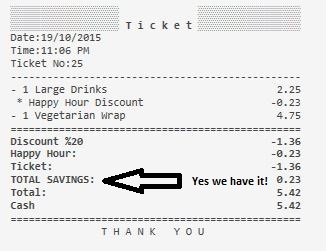With Brackets

``````[<J00>TOTAL SAVINGS:|[=F(-1*(TN('{ORDER TAG TOTAL:Discount}')+TN('{ORDER TAG TOTAL:VIP Discount}')+TN('{ORDER TAG TOTAL:HH Discount}'))+TN('{ORDER STATE TOTAL:Gift}'))]]
``````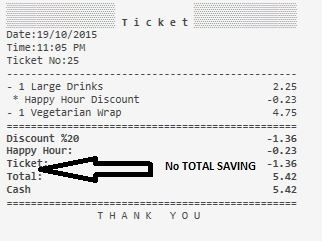Ok the line definitely has a 23c value so what is going on here?

That might be just a bug. Let me reproduce it on my side.

On my first try it appears fine when I have a gift in my ticket.

What could be different on your setup?

I think i have this issue too long time ago since V4 (square bracket inside square bracket). I just use ticket tag for total saving.